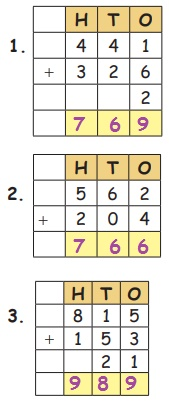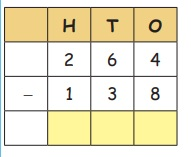Home | | Maths 3rd Std | Addition and Subtraction

## Chapter: 3rd Maths : Term 1 Unit 2 : Numbers

Frame the questions related to the given addition and subtraction facts

Recalla. 70 + 35 = 105

b. 68 + 23 = 91

c. 51 + 37 = 88

d. 55 + 18 = 73

e. 56 + 33 = 89

f. 57 + 33 = 90

g. 70 + 35 = 105

Addition of Three Digit Numbers (Without Regrouping)

Example : Add 132 and 241So using abacus, first put 132 as:

then add 241, as 2 more in hundred, 4 more in tens and 2 more in ones.Answer of addition of two or three numbers is called sum of the numbers.

sum = 373

Example : Add 342 + 515 + 12Sum = 869

Try this4. 34 + 452 + 3 = 489

Addition of Three Digit Numbers (With Regrouping)

Example : Add 556 and 1946 + 4 = 10 Ones = 1 Ten

With regrouping.

10 ones = 1 Ten + 0 ones

So, we put 0 in ones place and carry over 1 to ten place.1 + 5 + 9 = 15 tens

15 tens = 1 hundred + 5 tens

So, we put 5 in tens place. And carry over 1 to hundred place.1 + 5 + 1 = 7 hundred

So, we put 7 in hundreds place.

Sum = 750

Try thisd. 921 + 20 + 61 = 1002

e. 28 + 195 + 6 = 229

2. SUBTRACTION

Recall:

a. 45 – 35 = 10

b. 87 – 69 = 18

c. 49 – 38 = 11

d. 99 − 55 = 44Subtraction of Three Digit Numbers (Without Regrouping)

Example: Subtract 344 from 845Now, remove 3 in hundred, 4 in tens and 4 in ones as

Answer of subtraction of two numbers is called difference of the two numbers.

Difference = 501Example : Subtract 213 from 735Difference = 522

Try this

Subtract the following numbers.Subtraction of Three Digit Numbers (With Regrouping)

Example : Subtract 138 from 264Step : 1We cannot subtract 8 from 14. so regroup 1 ten into 10 ones.

148=6

Step : 253=2

Step : 321=1

Try this

Subtract the following numbers.Addition and subtraction by using standard algorithmExample:

Step : 15 + 6 = 11 ones,

11 ones = 1 tens + 1 one put 1 in ones place and carry over 1 to ten place.

Step : 21 + 7 + 3 = 11 tens ;

11 tens = 1 hundred + 1 tens. Put 1 in tens place and carry over 1 to hundred place.

Step : 31 + 6 + 1 = 8 hundreds put 8 in hundreds place

Puzzle : I am a 3 digit number. If you add 5 tens with me, I will become greatest 3 digit number Find me. 949

Teacher’s note : Teacher can help the children to do the Addition problems by using abacus kit.

Example :

Subtract 386 from 724Step :1

Subtract onesBorrow 1 ten from 2 tens then add to 4 ones we get 14 in one’s place.

146=8

Step : 2

Subtract tensBorrow 1 hundred from 7 hundreds then add to 1 ten we get 11 in ten’s place.

118=3

Step : 3

Subtract hundreds63=3

Difference = 338

Play with numbers : choose any 3 numbers Frame a 3− digit numbers and reverse it. Find their sum and difference. 642 : 642+246 = 888; 642-246=396

Teacher’s note : Teacher can help the children to do the Subtraction problems by using abacus kit.

Daily life situation involving addition and subtraction.

Example :

a. 452 Mangoes are grown in farm A and 349 in farm B. Find the total number of mangoes grown in both farms.Mangoes in farm A = 452

Mangoes in farm B = 349

Total number of Mangoes = 801

b. Amuthan saved rupees 125 on the first day and rupees 200 in the second day. find the total amount saved by him in two daysThe first day saving = 125

The second day saving = 200

Total saving = 325

c. Kumar earned rupees 800 in a day and spent rupees 450. Find the amount saved by him?

His one day income = 800

Amount spent = 450

Savings amount = 350

Frame questions for addition and subtraction for the picture below. one is done for you.Rani Chose 2 shirts from the hanger and 3 shirts from the rack. Find the total number of shirts chosen by him.

shirts from the hanger : 2

shirts from the rack : 3

2+3=5

Frame the questions related to the given addition and subtraction facts

Examples :

281 + 240 =

A dairy booth sells 281 bottles of milk on first day and 240 bottles of milk on second day. Find the total number of bottles sold on both the days.

352 148 = ?

There are 352 oranges on a tree 148 oranges were plucked from the tree. How many oranges are remaining in the tree ?

Try this

There were 10 egg trays each with 10 eggs in Valavan’s egg shop. He sold eggs in 3 trays and found that eggs in 2 trays were rotten. Find the number of eggs reamining in valavan’s shop.Total number of eggs in valavan’s shop = 100.

Number of eggs sold + number of eggs rotten = 30 + 20 = 50.

Number of eggs remaining in the shop = 50

Practice

Frame questions for the given addition and subtraction facts.

118+212=?

Mahendran has 118 pencils and 212 pens. How many items does he have in total?

717−515= ?

Mahesh has Rs. 715. She spent Rs. 515.Then what is the remaining money?

200+300=?

Anitha has 200 bombs and Karthi has 300 bombs. How many bombs do they have in total?

169 + 243 = ?

Savitha has 169 bananas and 243 custard apples. How many fruits does she have in total?

15050=?

MuthuMari has 150 orange, he sold 50 orange. How many orange does he have in total.

500355=?

Saminathan has Rs. 500, he gave Rs. 355 to his brother. How much amount does he have?

999199=?

Srinathan has 999 balls, he used 199 balls. How many balls does he have?

Tags : Numbers | Term 1 Chapter 2 | 3rd Maths , 3rd Maths : Term 1 Unit 2 : Numbers
Study Material, Lecturing Notes, Assignment, Reference, Wiki description explanation, brief detail
3rd Maths : Term 1 Unit 2 : Numbers : Addition and Subtraction | Numbers | Term 1 Chapter 2 | 3rd Maths